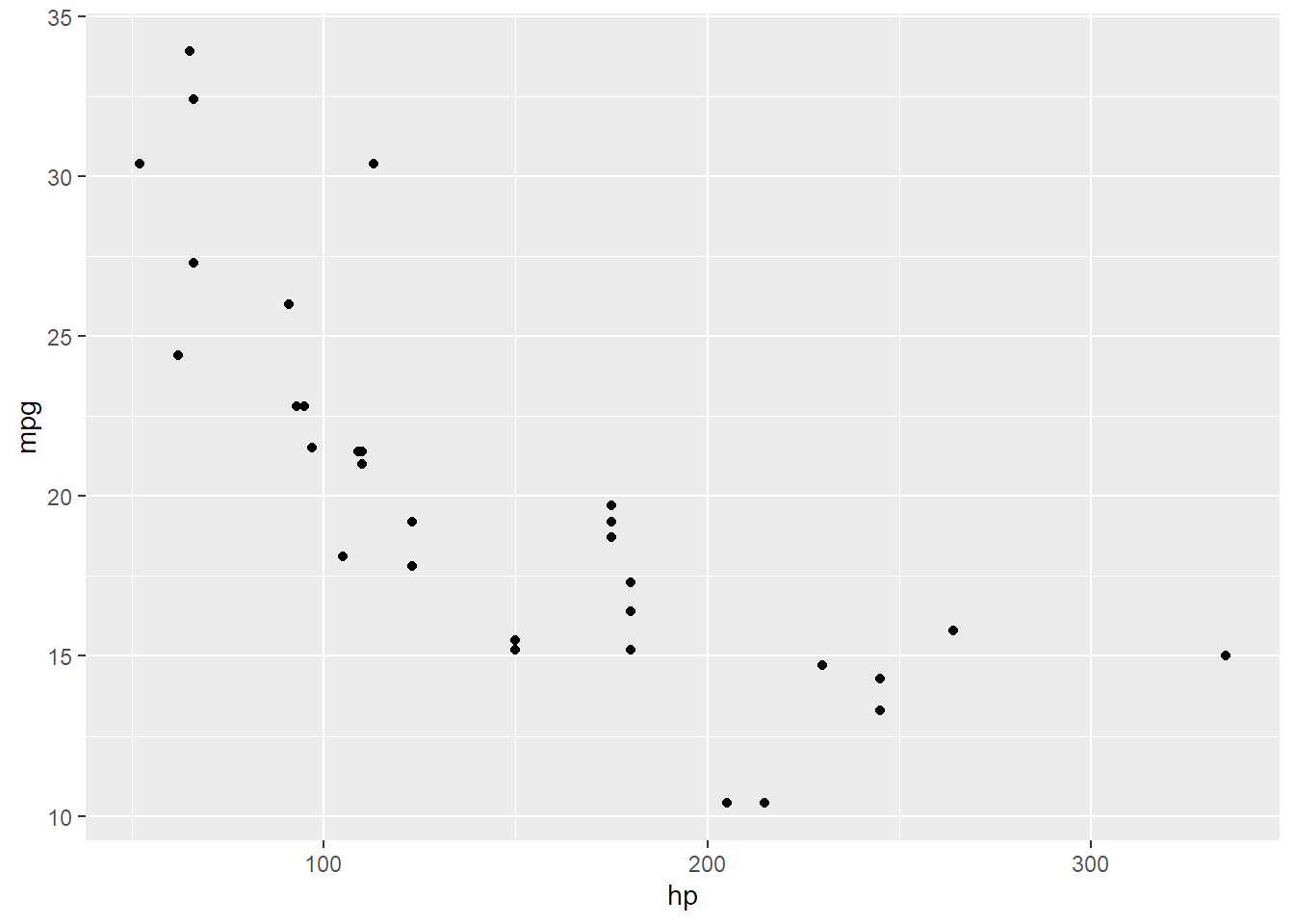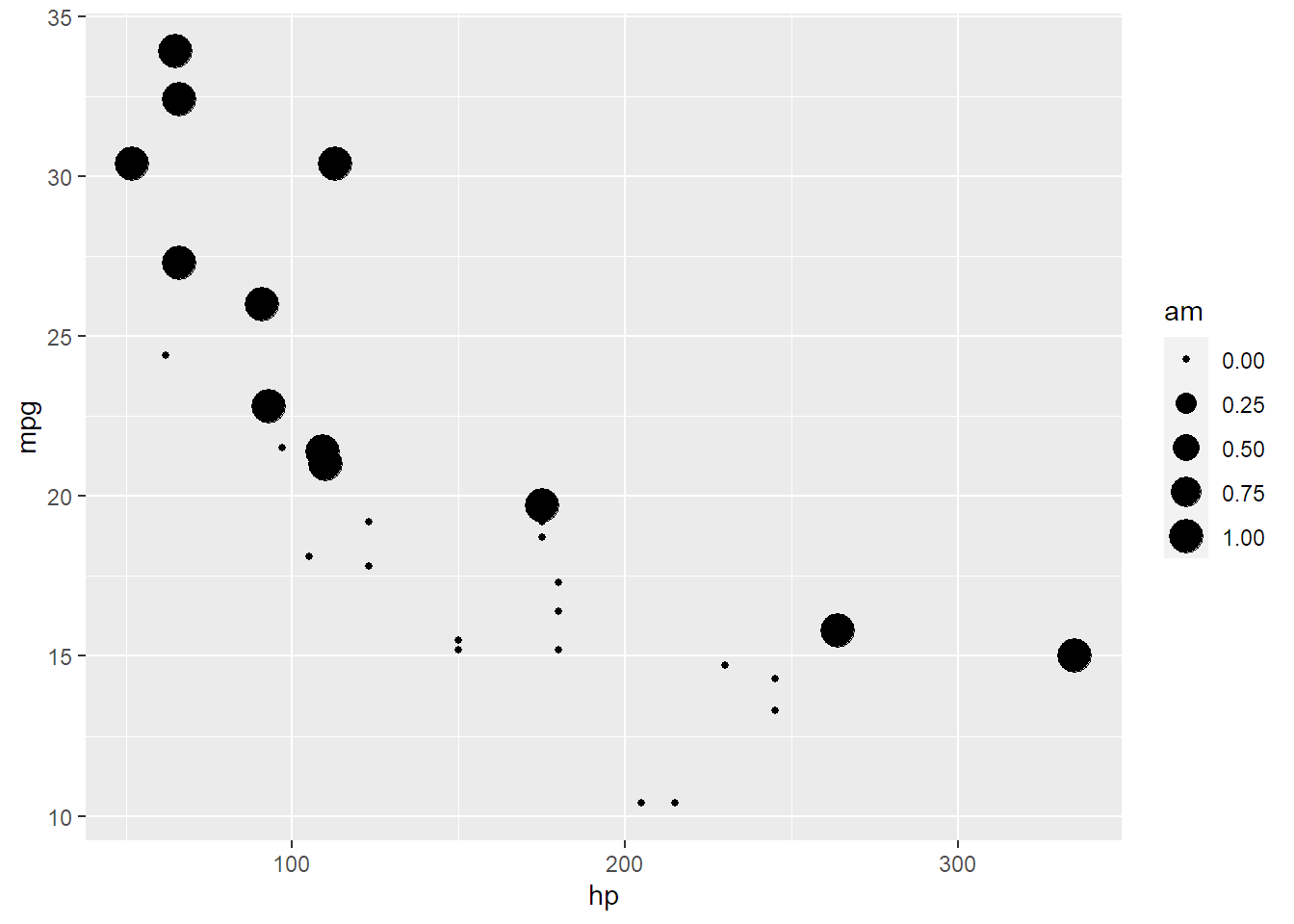## A function that creates ggplots

create_plot <- function(dataset, aesthetic){

ggplot(dataset) +
geom_point(aesthetic)

}

The function above takes a dataset and an aesthetic made using ggplot2::aes() to create a plot:

create_plot(mtcars, aes(y = mpg, x = hp))Let’s suppose that we want to generate a document that would look like this:

• first a section title, with the dataset used;
• then a plot

So it would look like this:

## Dataset used: “mtcars”

create_plot(mtcars, aes(y = mpg, x = hp))We don’t want to create these sections for every aesthetic by hand.

Instead, we can make use of a child document that gets knitted separately and then embedded in the parent document. The chunk below makes use of this trick:

x <- list(aes(y = mpg, x = hp),
aes(y = mpg, x = hp, size = am))

res <- lapply(x,
function(dataset, x){

knitr::knit_child(text = c(

'\n',
'## Dataset used: r deparse(substitute(dataset))',
'\n',
'{r, echo = F}',
'print(create_plot(dataset, x))',
''

),
envir = environment(),
quiet = TRUE)

}, dataset = mtcars)

cat(unlist(res), sep = "\n")

## Dataset used: mtcars## Dataset used: mtcarsThe child document is the text argument to the knit_child() function. text is literal R Markdown code: we define a level 2 header, and then an R chunk. This child document gets knitted, so we need to specify the environment in which it should get knitted. This means that the child document will get knitted in the same environment as the parent document (our current global environment). This way, every package that get loaded and every function or variable that got defined in the parent document will also be available to the child document.

To get the dataset name as a string, we use the deparse(substitute(dataset)) trick; this substitutes “dataset” by its bound value, so mtcars. But mtcars is an expression and we don’t want it to get evaluated, or the contents of the entire dataset would be used in the title of the section. So we use deparse() which turns unevaluated expressions into strings.

We then use lapply() to loop over two aesthetics with an anonymous function that encapsulates the child document. So we get two child documents that get knitted, one per aesthetic. This gets saved into variable res. This is thus a list of knitted Markdown.

Finally, we need unlist res to actually merge the Markdown code from the child documents into the parent document.